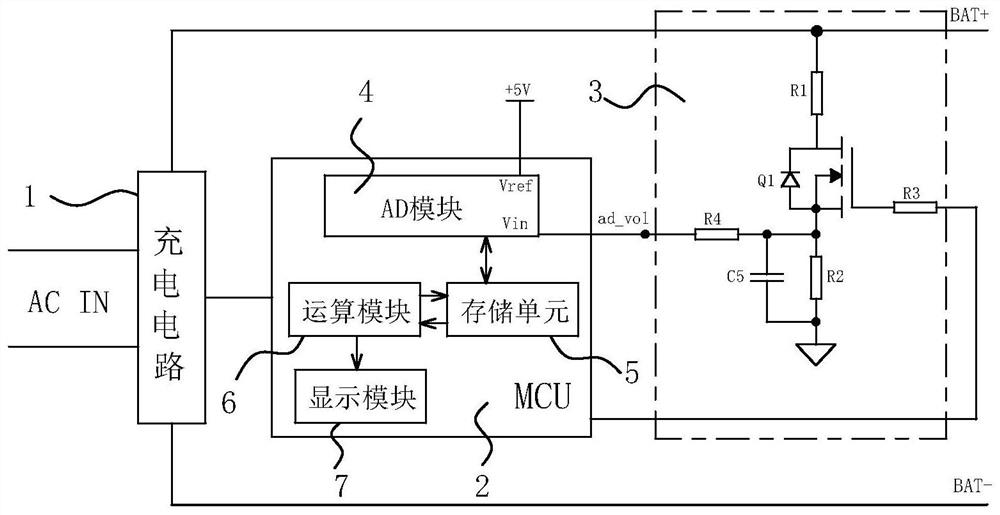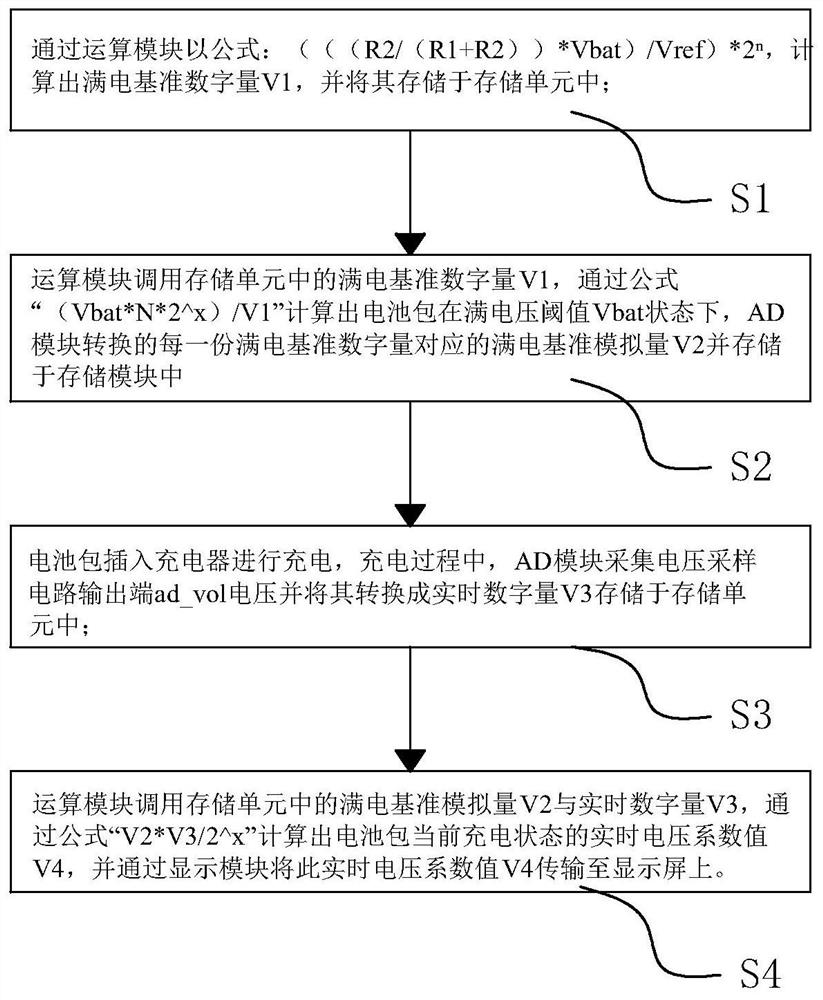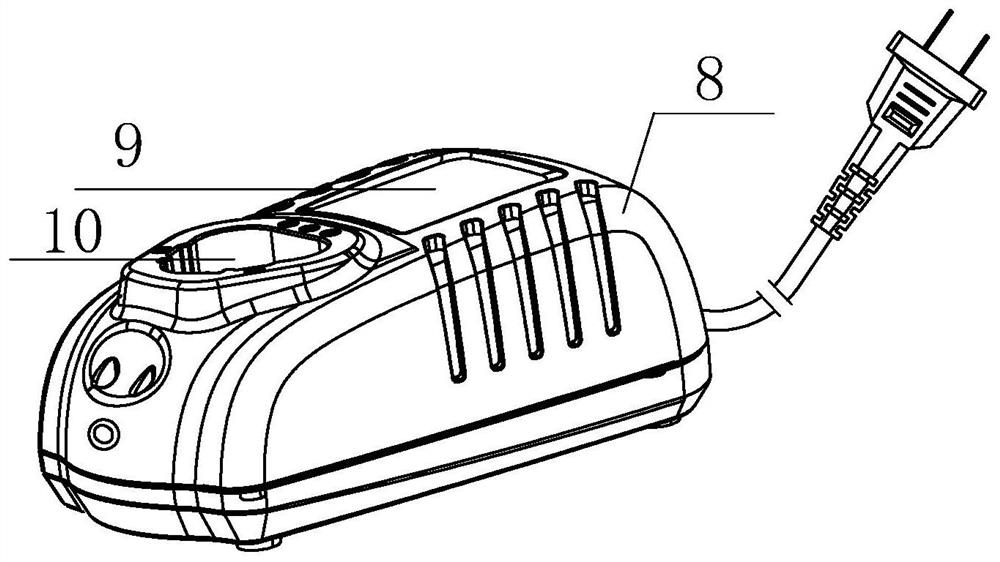# Method for detecting charging voltage of battery pack

## A charging voltage and detection method technology, applied in the field of electronics, can solve the problems that the battery pack is not full, the user cannot know the current power of the battery pack, and the circuit board lacks the voltage calibration function, etc., and achieves the effect of improving the sampling accuracy.

Pending Publication Date: 2021-12-28
JIANGSU DONGCHENG TOOLS TECH CO LTD
0 Cites 0 Cited by

## AI-Extracted Technical Summary

### Problems solved by technology

 In daily life, when the battery or battery pack or battery pack we use is exhausted, we need to use a charger to charge it. However, during the charging process of a general charger, there is no display on the charger housing, resulting in The user cannot know the current power of the battery pack; or there is a portable display on the charger shell, but because the circuit board in its own MCU controller lacks the voltage calibration function, the AD module converts the voltage analog value at the output of the voltage sampling circuit into After the digital quantity N1, directly compare it with the digital quantity N2 ...
the structure of the environmentally friendly knitted fabric provided by the present invention; figure 2 Flow chart of the yarn wrapping machine for environmentally friendly knitted fabrics and storage devices; image 3 Is the parameter map of the yarn covering machine
View more

## Abstract

The invention discloses a method for detecting charging voltage of a battery pack. The method is used for calibrating a voltage display numerical value of a charger, and comprises the following steps: firstly, calculating a full-power reference digital quantity V1 converted by an AD module when the battery pack is in a full-voltage threshold Vbat state by an operation module; secondly, calculating a full-power reference analog quantity V2 corresponding to each full-power reference digital quantity by an operation module; then enabling the AD module to collect a voltage real-time digital quantity V3; and finally, enabling the operation module to calculate a real-time voltage coefficient value V4 of charging of the battery pack, and displaying the real-time voltage coefficient value V4 on the display screen. An existing controller algorithm structure is changed, the real-time digital quantity V3 converted by the AD module is further calibrated through an algorithm formula in the operation module and converted into the real-time voltage coefficient value V4, then the value is displayed on the display screen, the real-time voltage coefficient value V4 can reflect the charging electric quantity of a current battery pack more visually and clearly, the real-time voltage coefficient value V4 can also reflect the charging electric quantity of the current battery pack more visually and clearly, and the voltage value of the battery pack is amplified by 100 times, so that the voltage display precision is further improved.

Application Domain

Technology Topic

## Image

•••## Examples

• Experimental program(1)

### Example Embodiment

 The terms used in the present invention are merely for the purpose of describing particular embodiments only, not intended to limit the present invention. The following example, "upper", "lower", "left", "right" indicating the orientation or positional relationship of the orientation or position terms are only based on the relationship shown in the drawings, for convenience of description only and the present invention is to simplify the description, and not indicate or imply that the circuit element referred to must have a particular orientation in a particular orientation or configuration and operation, it can not be construed as limiting the present invention.
 like Figure 1 to 3 A battery pack shown in the charging voltage detecting method for calibrating display charger voltage value, comprising the steps of:
 Step: power charger, its internal arithmetic module 2, the controller 6 calls the storage unit 5 in the pre-stored threshold battery voltage Vbat is full package, and to the reference voltage Vref 4 AD module as a reference, by a formula "(( (R2 / (R1 + R2)) * Vbat) / Vref) * 2 n "Calculate the battery pack at full voltage threshold value Vbat state 4 converts the AD module is fully charged reference digital V1 and stored in the storage unit 5, wherein full power reference digital quantity V1 to the third output terminal voltage sampling circuit ad_vol voltage analog after the amount of the AD module 4 after digital conversion;
 Step Two: 6 arithmetic module storage unit 5 calls the full digital power reference V1, by the formula "(Vbat * N * 2 x ) / V1 "calculates the reference analog fully charged battery pack at full voltage threshold value Vbat state, every full power reference digital AD module 4 corresponding to the converted V2 and stored in the storage unit, wherein the full power reference analog V2 is the amount of the full battery pack voltage threshold V1 Vbat into equal parts, single parts of full power analog reference each full charge amount corresponding to the reference number;
 The third step: inserting the battery pack charger for charging, the charging process, the AD acquisition module 4 3 ad_vol output of the voltage sampling circuit voltage and converting it to the real-time digital V3 stored in the storage unit 5;
 The fourth step: calculating a reference analog full power storage unit 5 calls the module 6 in the amount of real-time digital V2 and V3, by the formula "V2 * V3 / 2 x "Calculates the current state of charge of the battery pack based real-time voltage value V4, and through the display module 7 on this real-time voltage value V4 transmission system to the display screen 9, wherein the voltage coefficient values ​​in real time for real-time analog voltage V4 amplified N times quantity.
The charger includes a housing 8, a battery pack slot 10, and a display screen 9, a control circuit board mounted within the outer casing 8, the control circuit board including a charging circuit 1, which The controller 2 and the voltage sampling circuit 3 are electrically connected to the controller 2 and is positive, and the negative electrode terminal is electrically connected to the positive and negative terminal of the battery pack slot 10; the voltage sampling The circuit 3 input ad_bat is electrically connected to the positive terminal of the battery pack slot 10 and its output AD_VOL is electrically connected to the controller 2.
 The controller 2 includes the AD module 4, the memory cell 5 electrically connected to the AD module 4, and electrically connected to the memory cell 5 and electrically connected to the arithmetic module 6 The display module 7, the AD module 4 input terminal VIN is electrically connected to the output terminal AD_VOL of the voltage sampling circuit 3 for acquiring the output terminal AD_VOL voltage of the voltage sampling circuit 3 and converts it to real-time numbers. Quantity V3;
 The storage unit 5 is configured to store the battery pack full voltage threshold VBAT, the full-electrical reference digital volume V1, the full power reference analog quantity V2 and the real-time digital V3;
 The arithmetic module 6 is configured to calculate the full electrical reference digital V1, the full power reference analog quantity V2, and the real time voltage coefficient value V4, and transmit the real-time voltage coefficient value V4 signal to the display. Module 7;
 The display module 7 is electrically connected to the display screen 9, and the real-time voltage coefficient value V4 received by the display module is displayed by the display screen.
 The voltage sampling circuit 3 includes a voltage dividing resistor R1, a switching element Q1, a voltage resistor R2, and the upper end of the voltage resistor R1 is connected to the positive terminal of the battery pack slot 10, and the lower end is connected to the switching element Q1 drain Pole, the switching element Q1 source is connected to the upper end of the voltage resistor R2, the lower end of the voltage resistor R2, wherein the voltage at both ends of the voltage resistor R2 does not exceed 5V to prevent the MCU controller 2. Turned by it.
 The switching element Q1 gate is coupled to the controller 2, and the switching element Q1 is electrically connected at an input terminal Vin of the AD module 4 at an input terminal Vin of the AD module 4.
 The switching element Q1 gate and the controller are connected between the switching elements Q1 and the partial voltage resistor R2 connection to the Split resistance R4 between the AD module input terminal Vin, the shunt resistor The left end of the R4 is the voltage sampling circuit 3 output end ad_vol.
 The formula "((((R2 / (R1 + R2)) * VBAT) / VREF) * 2N" is the conversion accuracy of the AD module 4; the formula (VBAT * N * 2 x ) / V1 "and the formula" V2 * V3 / 2 x "The N is the amplification factor of the battery pack full voltage threshold VBAT, X is an enlarged accuracy of the battery pack full voltage threshold VBAT.
 The magnification of the magnification N number is 10, and the value is 100, and the purpose is to improve the display accuracy of the battery pack real-time voltage, accurate to millivolts.
 The conversion accuracy N of the AD module 4 is 12bit, the reference voltage Vref 5 V, the controller 2 employ the MCU controller.
 The magnifying accuracy x ≥ 10, facilitates further increase the calculation accuracy of the full power reference analog value V2 and the real-time voltage coefficient value V4, wherein the calculation accuracy is 2 x In order to facilitate the calculation of the internal chip of the controller.
 The working principle of the present invention is:
 (1) Positive derivation: The battery is unknown, with R1 = 510K, R2 = 51K, VBAT = 21V, N = 12, x = 10, n = 100, VREF = 5V, V3 = 819.2 as an example:
 S1: The full-electric reference digital V1 is 1563 and stored in the storage unit 5 by the operation module 6: ((((R2 / (R1 + R2)) * VBAT) / VREF) * 2n calculated.
 S2: Through the arithmetic module 6 with formula: (vbat * n * 2 x ) / V1, calculate the full-electric reference analog quantity V2 1375 and stored in the storage unit 5, where the VBAT multiplied by N indicating the VBAT value by 100 times, obtain the voltage coefficient value of 2100, and multiplied by 2 x Indicates that the voltage is further enlarged to improve the calculation accuracy of the full power reference analog amount V2.
 S3: Insert the battery pack into the charger, during charging, the AD module 4 acquires the voltage sampling circuit 3 output terminal AD_VOL voltage and converts it to real-time digital volume V3 = 819.2, and stored in storage unit 5;
 S4: Operation Module 6 Call the full power reference analog V2 and real-time digital V3 in the storage unit 5, by formula "V2 * V3 / 2 x "Calculate the real-time voltage coefficient value V4 = 1100 of the current charging state of the battery pack, and transmit this real-time voltage coefficient value 1100 through the display module 7 to the display screen 9, the real-time voltage coefficient value V4 is an enlarged 100-fold real-time voltage simulation. The amount, that is, the real-time voltage of the battery pack charge is 11V.
 Among them, formula "V2 * V4 / 2 x "The meaning of V2 * V4 is: 819.2 Voltage simulation corresponding to the full power reference digital, then divided by 2 x , Meaning to the magnification accuracy 2 in step S2 in step S2 x Restore, get a real-time voltage coefficient value V4 amplified by 100 times.
 (2) Reverse derivation: It is known that the battery has a real-time voltage of 11V, with R1 = 510K, R2 = 51K, VBAT = 21V, N = 12, x = 10, n = 100, VREF = 5V as an example:
 S1: V1 = ((((R2 / (R1 + R2)) * VBAT) / VREF) * 2N = 1563;
 S2: V2 = (vbat * n * 2 x ) / V1 = 1375;
 S3: When the battery pack is charged, the AD module 4 acquires the voltage sampling circuit output end AD_VOL voltage simulation value: (R2 / (R1 + R2)) * 11 = 1V, convert it into real-time digital V3 = (1/5 )*2 12 = 819.2 and stored in the storage unit 5;
 S4: v4 = "v2 * v3 / 2 x "= 1100, at which point the number shown on the display 9 is 1100, that is, the real-time voltage of the battery pack charge is 11V.
 (3) Deflected: According to V1 = (((R2 / (R1 + R2)) * VBAT) / VREF) * 2 n , V2 = (vbat * n * 2 x ) / V1, v3 = (((R2 / (R1 + R2)) * V solid) / VREF) * 2 n , Seeking V4 = V2 * V3 / 2 x The value, the formula is simple to make V4 = V real * n, that is, the final display shows the real-time voltage coefficient value V4 after enlargement N times.
 The present invention passes through the algorithm structure of the existing charger, and the real-time digital V3 of the AD module conversion when the battery pack is further calibrated by the algorithm formula in the calculation module 6, and the real-time digital V3 is converted into the current battery pack. The real-time voltage coefficient value V4 at the time of charging, eventually presented the real-time voltage coefficient value V4 on display module 7, which can not only be more intuitive, clearly reflecting the charging power of the current battery pack, but also The battery pack voltage value is 100 times to further improve the sampling accuracy of the circuit.
 The present invention is not limited to the above specific embodiments, and those skilled in the art can be readily understood that there are many other replacement methods of the battery pack charging voltage of the present invention without departing from the principles and category of the present invention. According to the scope of the invention, the scope of the invention is subject to the content of the claims.
the structure of the environmentally friendly knitted fabric provided by the present invention; figure 2 Flow chart of the yarn wrapping machine for environmentally friendly knitted fabrics and storage devices; image 3 Is the parameter map of the yarn covering machine

## PUM## Description & Claims & Application Information

We can also present the details of the Description, Claims and Application information to help users get a comprehensive understanding of the technical details of the patent, such as background art, summary of invention, brief description of drawings, description of embodiments, and other original content. On the other hand, users can also determine the specific scope of protection of the technology through the list of claims; as well as understand the changes in the life cycle of the technology with the presentation of the patent timeline. Login to view more.
the structure of the environmentally friendly knitted fabric provided by the present invention; figure 2 Flow chart of the yarn wrapping machine for environmentally friendly knitted fabrics and storage devices; image 3 Is the parameter map of the yarn covering machine

## Protector for three-phase motor of alternating-current or direct-current electric locomotive

Owner:ZHUZHOU CSR TIMES ELECTRIC CO LTD

## Analog accelerometer output signal transmission device

InactiveCN103389091AImprove sampling accuracySolve the problem of easy introduction of electromagnetic interference signalsNavigation by speed/acceleration measurementsVIT signalsEngineering
Owner:西安应用光学研究所

## High-efficiency energy-saving current amplifier

Owner:NORTH CHINA ELECTRIC POWER UNIV (BAODING)

Owner:BYD CO LTD

## Method and apparatus for identifying high-risk commodities by sampling inspection

InactiveCN109146585AReduce sampling costImprove sampling accuracyBuying/selling/leasing transactionsLimit valueComputer science
Owner:BEIJING JINGDONG SHANGKE INFORMATION TECH CO LTD +1

## Classification and recommendation of technical efficacy words

• Improve sampling accuracy

Owner:苏向东

Owner:ZTE CORP

## Human energy metabolism detection device and method

InactiveCN104665835AImprove sampling accuracyThe sampling process is uninterruptedDiagnostic recording/measuringSensorsCarbon dioxide sensorThrottle
Owner:HEFEI INSTITUTES OF PHYSICAL SCIENCE - CHINESE ACAD OF SCI +1

## Method of nondestructive testing with digital shearing speckle interferometry and device thereof

Owner:NORTHWESTERN POLYTECHNICAL UNIV

## Solid recorder for posture measuring

InactiveCN101261748AImprove sampling accuracySampling rate adjustableRegistering/indicating working of vehiclesSolid state recorderLinear acceleration
Owner:ZHONGBEI UNIV
Who we serve
• R&D Engineer
• R&D Manager
• IP Professional
Why Eureka